Download Presentation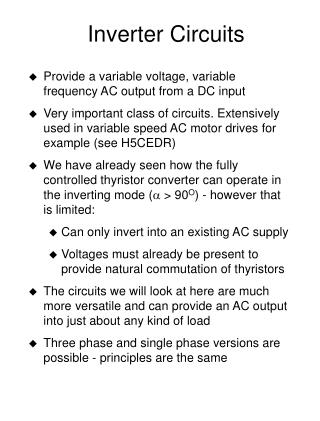Inverter Circuits

# Inverter Circuits - PowerPoint PPT Presentation

Inverter Circuits. Provide a variable voltage, variable frequency AC output from a DC input Very important class of circuits. Extensively used in variable speed AC motor drives for example (see H5CEDR)I am the owner, or an agent authorized to act on behalf of the owner, of the copyrighted work described.
Download Presentation## Inverter Circuits

An Image/Link below is provided (as is) to download presentation

Download Policy: Content on the Website is provided to you AS IS for your information and personal use and may not be sold / licensed / shared on other websites without getting consent from its author.While downloading, if for some reason you are not able to download a presentation, the publisher may have deleted the file from their server.

- - - - - - - - - - - - - - - - - - - - - - - - - - E N D - - - - - - - - - - - - - - - - - - - - - - - - - -
Presentation Transcript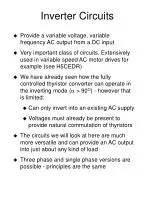Inverter Circuits
• Provide a variable voltage, variable frequency AC output from a DC input
• Very important class of circuits. Extensively used in variable speed AC motor drives for example (see H5CEDR)
• We have already seen how the fully controlled thyristor converter can operate in the inverting mode ( > 90O) - however that is limited:
• Can only invert into an existing AC supply
• Voltages must already be present to provide natural commutation of thyristors
• The circuits we will look at here are much more versatile and can provide an AC output into just about any kind of load
• Three phase and single phase versions are possible - principles are the same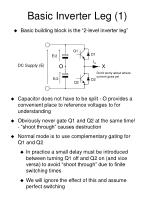Q1

D1

E/2

IAC

DC Supply (E)

O

X

Don’t worry about where

current goes yet

E/2

D2

Q2

Basic Inverter Leg (1)
• Basic building block is the “2-level inverter leg”
• Capacitor does not have to be split - O provides a convenient place to reference voltages to for understanding
• Obviously never gate Q1 and Q2 at the same time! - “shoot through” causes destruction
• Normal mode is to use complementary gating for Q1 and Q2
• In practice a small delay must be introduced between turning Q1 off and Q2 on (and vice versa) to avoid “shoot through” due to finite switching times
• We will ignore the effect of this and assume perfect switching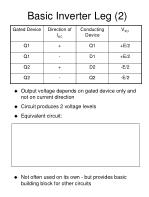Basic Inverter Leg (2)
• Output voltage depends on gated device only and not on current direction
• Circuit produces 2 voltage levels
• Equivalent circuit:
• Not often used on its own - but provides basic building block for other circuits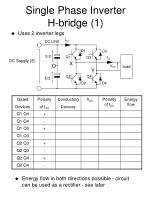IDC

D1

D3

E/2

Q1

Q3

IAC

DC Supply (E)

X

O

VAC

Y

Q2

Q4

E/2

D2

D4

Single Phase Inverter H-bridge (1)
• Uses 2 inverter legs
• Energy flow in both directions possible - circuit can be used as a rectifier - see later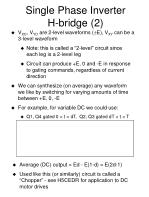Single Phase Inverter H-bridge (2)
• VXO, VYO are 2-level waveforms (E), VXY can be a 3-level waveform
• Note: this is called a “2-level” circuit since each leg is a 2-level leg
• Circuit can produce +E, 0 and -E in response to gating commands, regardless of current direction
• We can synthesize (on average) any waveform we like by switching for varying amounts of time between +E, 0, -E
• For example, for variable DC we could use:
• Q1, Q4 gated 0 < t < dT, Q2, Q3 gated dT < t < T
• Average (DC) output = Ed - E(1-d) = E(2d-1)
• Used like this (or similarly) circuit is called a “Chopper” - see H5CEDR for application to DC motor drives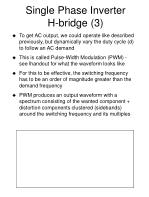Single Phase Inverter H-bridge (3)
• To get AC output, we could operate like described previously, but dynamically vary the duty cycle (d) to follow an AC demand
• This is called Pulse-Width Modulation (PWM) - see lhandout for what the waveform looks like
• For this to be effective, the switching frequency has to be an order of magnitude greater than the demand frequency
• PWM produces an output waveform with a spectrum consisting of the wanted component + distortion components clustered (sidebands) around the switching frequency and its multiples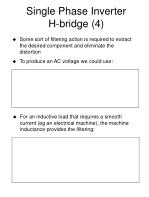Single Phase Inverter H-bridge (4)
• Some sort of filtering action is required to extract the desired component and eliminate the distortion
• To produce an AC voltage we could use:
• For an inductive load that requires a smooth current (eg an electrical machine), the machine inductance provides the filtering: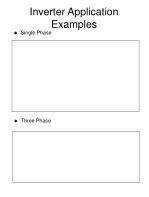Inverter Application Examples
• Single Phase
• Three Phase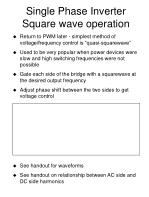Single Phase Inverter Square wave operation
• Return to PWM later - simplest method of voltage/frequency control is “quasi-squarewave”
• Used to be very popular when power devices were slow and high switching frequencies were not possible
• Gate each side of the bridge with a squarewave at the desired output frequency
• Adjust phase shift between the two sides to get voltage control
• See handout for waveforms
• See handout on relationship between AC side and DC side harmonics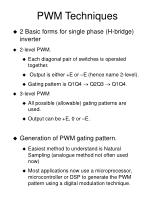PWM Techniques
• 2 Basic forms for single phase (H-bridge) inverter
• 2-level PWM.
• Each diagonal pair of switches is operated together.
• Output is either +E or –E (hence name 2-level).
• Gating pattern is Q1Q4  Q2Q3  Q1Q4.
• 3-level PWM
• All possible (allowable) gating patterns are used.
• Output can be +E, 0 or –E.
• Generation of PWM gating pattern.
• Easiest method to understand is Natural Sampling (analogue method not often used now)
• Most applications now use a microprocessor, microcontroller or DSP to generate the PWM pattern using a digital modulation technique.Natural Sampling 1
• See handout for detail of comparison process
• Definitions:Natural Sampling 2
• Frequency ratio (FR) can be integer (synchronous PWM) or non-integer (asynchronous PWM).
• It is normal now to keep the carrier frequency fixed as the modulating frequency is varied – hence most PWM today is asynchronous.
• Modulation Index (MI) tells us how large the modulating frequency component at the inverter output will be for a given DC link voltage.
• Modulation Depth (MD) tells us how much we have modulated the pulses by (compared to an unmodulated 50% duty cycle carrier frequency squarewave).
• For Natural Sampling MI = MD (provided MD < 1)
• Hence control of amplitude and frequency of the modulating wave, provides direct frequency and voltage control at the inverter output.
• Spectrum of 2-level PWM: Modulating component + sidebands around carrier frequency + sidebands around 2 times carrier frequency etc – see HandoutNatural Sampling 3
• 3-level  use the same carrier for both sides of the H-bridge, but invert the modulating wave (180O shift).
• VXO and VYO are 2-level, VXY is 3-level.
• Components clustered as sidebands around odd multiples of the carrier frequency are in-phase in VXO and VYO and therefore cancel in VXY
• Other components are in anti-phase in VXO and VYO and therefore add in VXY
• 3-level produces less distortion for given carrier (switching) frequency – see HandoutDigital PWM
• Natural sampling is not suitable for a microprocessor implementation.
• Switching instants occur at the natural intersection between a triangle wave and a sinewave.
• Equation determining the switching instants has no analytical solution (transcendental equation) and can only be solved by iteration – no good for real time calculation.
• Microprocessor implementation uses the Regular Sampling method (or something similar).
• There are no continuous modulating or carrier waves.
• Time is divided into a sequence of carrier periods of width TC.
• The modulating wave exists as a series of samples, sampled either every TC (symmetric PWM) or every TC/2 (asymmetric PWM).
• One pulse is produced within each carrier period.
• Pulsewidth depends on either one sample of the modulating wave (symmetric PWM) or two samples of the modulating wave (asymmetric PWM).Regular SamplingSymmetric PWM
• Let SK-1, SK, SK+1 etc be the samples of the modulating wave sampled at rate (1/TC).
• Assume the modulating wave is scaled so that its peak amplitude is unity.
• Simple equations define the pulsewidths – OK for real time digital implementation.
• MD  MI for regular samplingRegular Samplingasymmetric PWM
• Let SAK-1, SBK-1, SAK, SBK, SAK+1, SBK+1 etc. be the samples of the modulating wave sampled at rate (2/TC).
• Assume the modulating wave is scaled so that its peak amplitude is unity.
• Asymmetric PWM produces less distortion than symmetric PWM for a given carrier (switching frequency)
• MD  MI as for symmetric samplingPWM Miscellaneous
• Choice of carrier frequency
• Compromise depending on switching losses in the inverter and output waveform distortion.
• Also depends on the switching device technology used.
• Typical values: 16kHz (1kW), 5kHz (100kW), 1kHz (1MW) – assuming IGBT devices.
• Other types of PWM (not a complete list)
• Space Vector PWM
• Similar to regular sampling, but derived from the “space-phasor” representation of 3-phase quantities. Popular in “Vector controlled” induction motor drives (see H54IMD)
• “Optimised PWM”
• Spectrum of PWM is defined mathematically in terms of the pulsewidths. Numerical techniques are then used to calculate the pulsewidths to meet a particular performance target.
• For example: eliminate certain harmonics, minimise weighted sum of harmonics etc.
• Not popular except in some special applicationsDC Supply (E)

O

A

B

C

3-phase Inverter
• VAO etc are 2-level (±E/2), VAB etc are 3-level (±E and 0).
• Each leg is modulated using the same carrier, but with modulating waves 120o apart (3-phase).
• The large carrier frequency component in VAO etc cancels in VAB etc.
• PWM control of inverter gives variable voltage and variable frequency output.
• Average power flow can be bidirectional if the DC source can accept power input.3-phase AC to AC(rectifier - inverter)

RECTIFIER

INVERTER

3-PHASE

SUPPLY

3-Phase